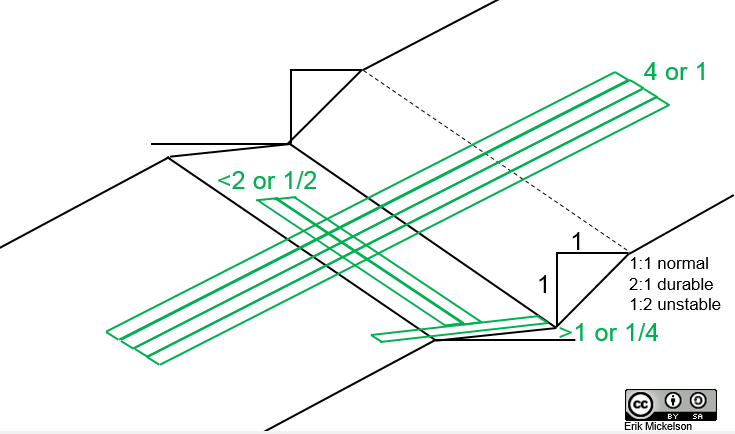Trailism

# Trail Math

A short and incomplete list of equations and calculators useful for trails.

#### Layout Equations

$latex \%Slope=\frac{Rise}{Run}\times100&s=2$

$latex Slope\textdegree=(\frac{Rise}{Run})\tan^{-1}&s=2$

or $latex Slope\textdegree=(\frac{\theta\%}{100})\tan^{-1}&s=2$

$latex Rise\:or\:Fall=b=(Running\:Grade)\sin\theta\textdegree&s=2$

note: Forest Service Trail Accessibility Guidelines (FSTAG) 7.4.3.2 “The cross slope [out slope] shall not exceed 1:20 (5 percent). Where the surface is paved or is elevated above the natural ground, the cross slope shall not be steeper than 1:48 (2 percent).”

### Half Rulein actuality the half rule is really equal to and less than…but it’s really not a “rule” as much as a suggestion

$latex Half\;Rule=Slope\;Grade\leqslant\frac{Fall\;Line}{2}&s=2$

$latex OutSlope\;Half\;Rule=OutSlope\;Grade\geqslant\frac{Fall\;Line}{4}&s=2$

or $latex OutSlope\;Half\;Rule=OutSlope\;Grade\geqslant\frac{Running\;Grade}{2}&s=2$

### Trail Length

A rough estimate of the trail length for a segment based on elevation gain or loss (typically the average grade should be less than 10%):

$latex Trail\;Distance=\frac{Elevation\;Change}{Desired\;\%Grade\;as\;decimal}&s=2$

### Turns

$latex 180\textdegree\;Turn\;Length= Circumference\;/2=(2\pi\times r)/2&s=2$

$latex 180\textdegree\;Turn\;Grade= (\%Slope\;Turn\;Descends/100)tan^{-1})/(\pi/2)&s=2$

180° Turn Grade? Rise/Run @ Diameter vs. Rise/Run @ Circumference:

#### More on the complicated machinations of turning on a bike:

I hope to publish some nomograms in the future for some of the above math applications on this and other pages.

Scroll to Top